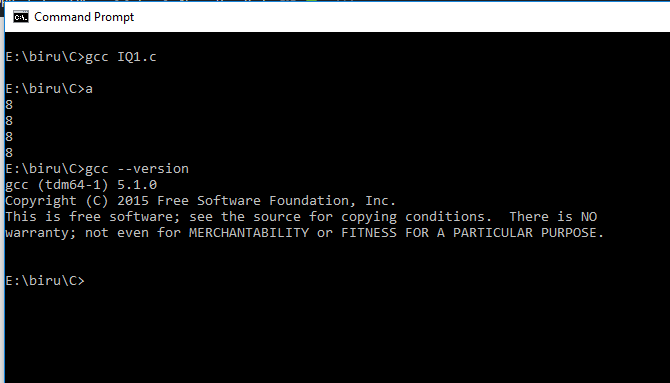Home ProgrammingC Language Size of Pointer-C Programming Interview Question

# Size of Pointer-C Programming Interview Question

1423 views

The main Objective of this interview question is to suggest you better concept of Size of Pointer. Either you are a learner of even an expert this article will enhance your knowledge in C. Size of Pointer is not much highlighted topic in various courses provided by universities and institutes, So this is very mush asked interview question in C programming language.

### Question:-

What will be the output of following code snippet?

```void main(){
int *p;
float *q;
double *r;
printf("%dn",sizeof(p));
printf("%dn",sizeof(q));
printf("%dn",sizeof(r));
printf("%d",sizeof(char*));
getch();
}```

If You are here to read this, I’m sure that you must be familiar with concept of pointer. Although I want to suggest little information about Pointers.

Pointers are the variables just like other variables but these can only store the address of memory location. Just like an integer variable can store an integer value and a character variable can store a character value only. Now We are clear that pointers store the memory address.

Now last thing is how large is the address of memory blocks used in our program. It depends on the compiler. That is if compiler is 8 bit then memory address is 8 bit long, if your compiler is 16 bit then memory address will be 16 bit long and so on. Now here In my case my compiler is 64 bit. So In my system memory address will be 64 bit long.

As we know Regardless of type all the pointers only store the address of memory location, The size of memory occupied by each of the pointers in above program is 64 bit. So This program will print 8888 as output Because 64 bit is equals to 8 byte.

So we can conclude that any pointer occupy the same amount of memory regardless of the type of pointer.

I am using gcc (tdm64-1) compiler it is 64 bit compiler. So i’m getting 8 byte as Size of Pointer. If you use 32 bit compiler you will get 4 as the Size of Pointer.